15分钟带你了解前端工程师必知的javascript设计模式(附详细思维导图和源码)徐小夕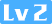1044 0 0

• 单例模式
• 构造器模式
• 建造者模式
• 代理模式
• 外观模式
• 观察者模式
• 策略模式
• 迭代器模式

## 正文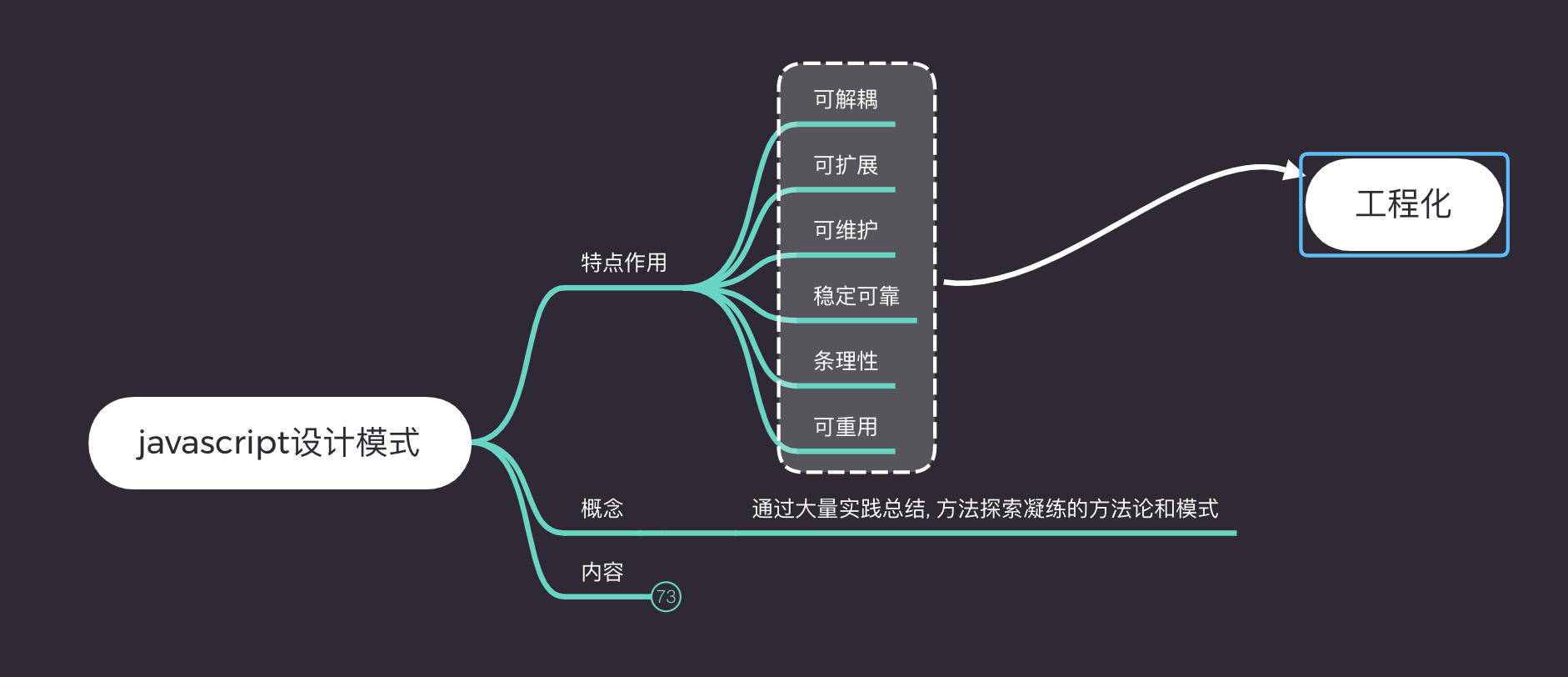以上笔者主要总结了几点使用设计模式能给工程带来的好处, 如代码可解耦, 可扩展性, 可靠性, 条理性, 可复用性. 接下来来看看我们javascript的第一个设计模式.

### 1. 单例模式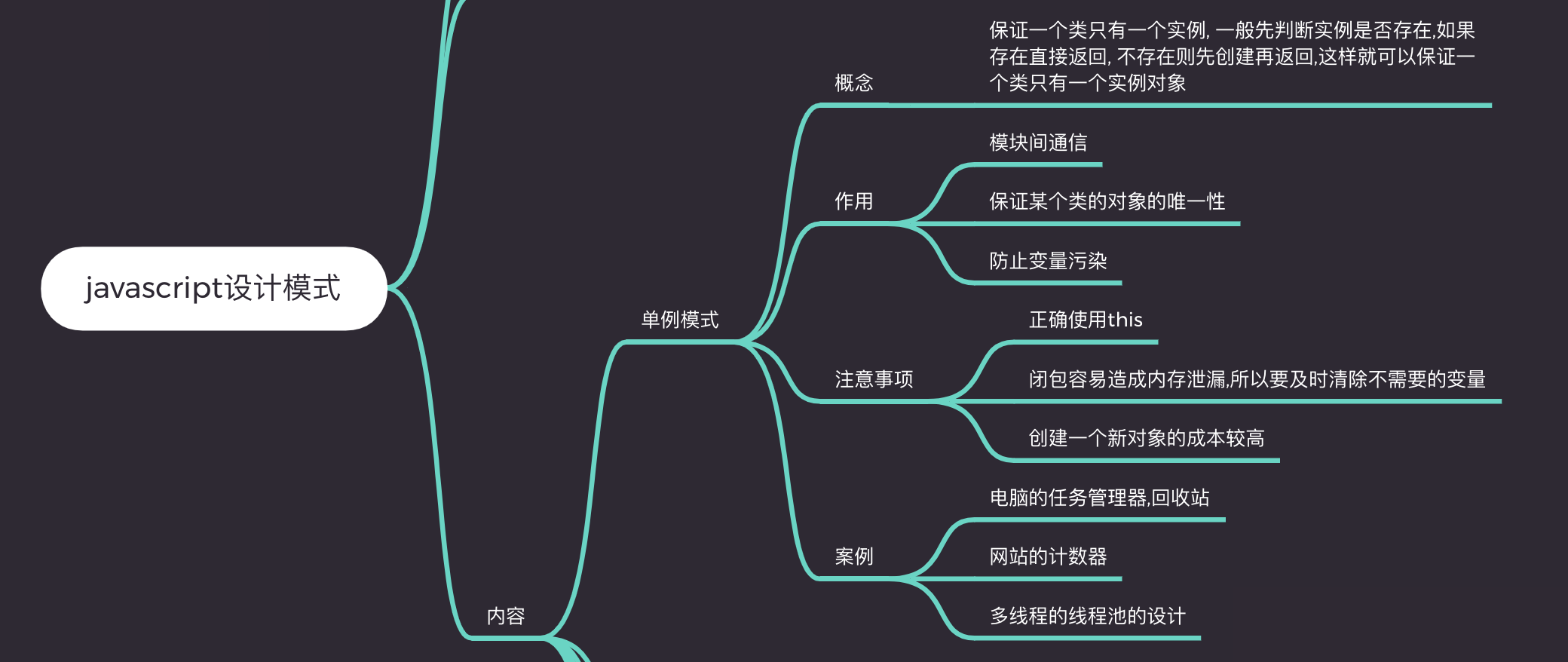• 模块间通信
• 保证某个类的对象的唯一性
• 防止变量污染

#### 1.3 注意事项

• 正确使用this
• 闭包容易造成内存泄漏,所以要及时清除不需要的变量
• 创建一个新对象的成本较高

#### 1.5 代码实现

``````(function(){
// 养鱼游戏
let fish = null
function catchFish() {
// 如果鱼存在,则直接返回
if(fish) {
return {
fish,
water: function() {
let water = this.fish.getAttribute('weight')
this.fish.setAttribute('weight', ++water)
}
}else {
// 如果鱼不存在,则获取鱼再返回
fish = document.querySelector('#cat')
return {
fish,
water: function() {
let water = this.fish.getAttribute('weight')
this.fish.setAttribute('weight', ++water)
}
}
}
}

// 每隔3小时喂一次水
setInterval(() => {
catchFish().water()
}, 3*60*60*1000)
})()``````

### 2. 构造器模式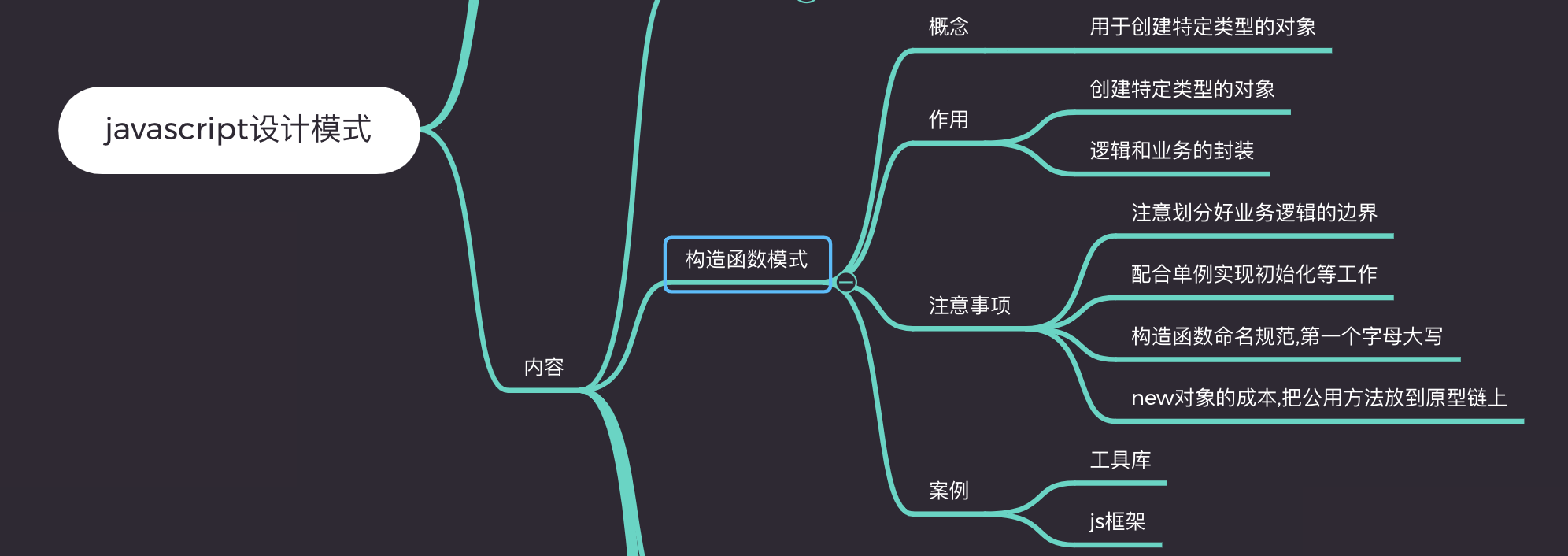• 创建特定类型的对象
• 逻辑和业务的封装

#### 注意事项

• 注意划分好业务逻辑的边界
• 配合单例实现初始化等工作
• 构造函数命名规范,第一个字母大写
• new对象的成本,把公用方法放到原型链上

#### 代码展示

``````function Tools(){
if(!(this instanceof Tools)){
return new Tools()
}
this.name = 'js工具库'
// 获取dom的方法
this.getEl = function(elem) {
return document.querySelector(elem)
}
// 判断是否是数组
this.isArray = function(arr) {
return Array.isArray(arr)
}
// 其他通用方法...
}``````

### 3. 建造者模式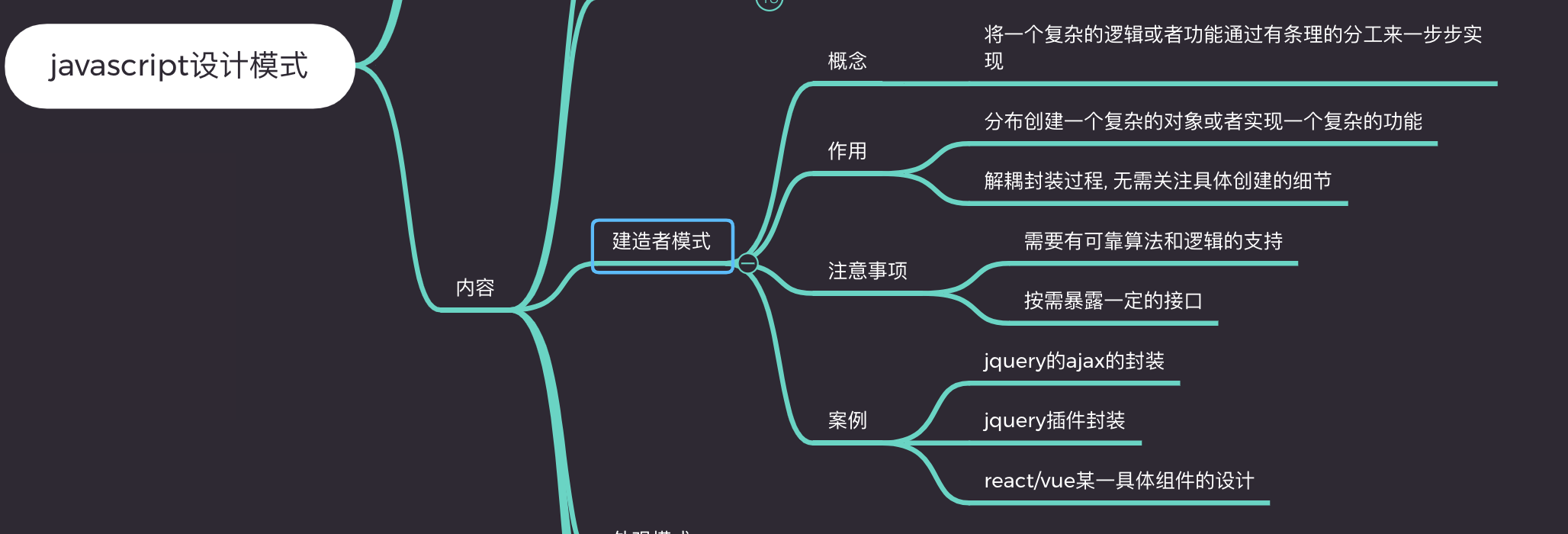#### 作用

• 分布创建一个复杂的对象或者实现一个复杂的功能
• 解耦封装过程, 无需关注具体创建的细节

#### 注意事项

• 需要有可靠算法和逻辑的支持
• 按需暴露一定的接口

#### 实际案例

• jquery的ajax的封装
• jquery插件封装
• react/vue某一具体组件的设计

#### 代码展示

``````// canvas绘制图形验证码
(function(){
function Gcode(el, option) {
this.el = typeof el === 'string' ? document.querySelector(el) : el;
this.option = option;
this.init();
}
Gcode.prototype = {
constructor: Gcode,
init: function() {
if(this.el.getContext) {
isSupportCanvas = true;
var ctx = this.el.getContext('2d'),
// 设置画布宽高
cw = this.el.width = this.option.width || 200,
ch = this.el.height = this.option.height || 40,
textLen = this.option.textLen || 4,
lineNum = this.option.lineNum || 4;
var text = this.randomText(textLen);

this.onClick(ctx, textLen, lineNum, cw, ch);
this.drawLine(ctx, lineNum, cw, ch);
this.drawText(ctx, text, ch);
}
},
onClick: function(ctx, textLen, lineNum, cw, ch) {
var _ = this;
text = _.randomText(textLen);
_.drawLine(ctx, lineNum, cw, ch);
_.drawText(ctx, text, ch);
}, false)
},
// 画干扰线
drawLine: function(ctx, lineNum, maxW, maxH) {
ctx.clearRect(0, 0, maxW, maxH);
for(var i=0; i < lineNum; i++) {
var dx1 = Math.random()* maxW,
dy1 = Math.random()* maxH,
dx2 = Math.random()* maxW,
dy2 = Math.random()* maxH;
ctx.strokeStyle = 'rgb(' + 255*Math.random() + ',' + 255*Math.random() + ',' + 255*Math.random() + ')';
ctx.beginPath();
ctx.moveTo(dx1, dy1);
ctx.lineTo(dx2, dy2);
ctx.stroke();
}
},
// 画文字
drawText: function(ctx, text, maxH) {
var len = text.length;
for(var i=0; i < len; i++) {
var dx = 30 * Math.random() + 30* i,
dy = Math.random()* 5 + maxH/2;
ctx.fillStyle = 'rgb(' + 255*Math.random() + ',' + 255*Math.random() + ',' + 255*Math.random() + ')';
ctx.font = '30px Helvetica';
ctx.textBaseline = 'middle';
ctx.fillText(text[i], dx, dy);
}
},
// 生成指定个数的随机文字
randomText: function(len) {
var source = ['a', 'b', 'c', 'd', 'e',
'f', 'g', 'h', 'i', 'j',
'k', 'l', 'm', 'o', 'p',
'q', 'r', 's', 't', 'u',
'v', 'w', 'x', 'y', 'z'];
var result = [];
var sourceLen = source.length;
for(var i=0; i< len; i++) {
var text = this.generateUniqueText(source, result, sourceLen);
result.push(text)
}
return result.join('')
},
// 生成唯一文字
generateUniqueText: function(source, hasList, limit) {
var text = source[Math.floor(Math.random()*limit)];
if(hasList.indexOf(text) > -1) {
return this.generateUniqueText(source, hasList, limit)
}else {
return text
}
}
}
new Gcode('#canvas_code', {
lineNum: 6
})
})();
// 调用
new Gcode('#canvas_code', {
lineNum: 6
})``````

### 4. 代理模式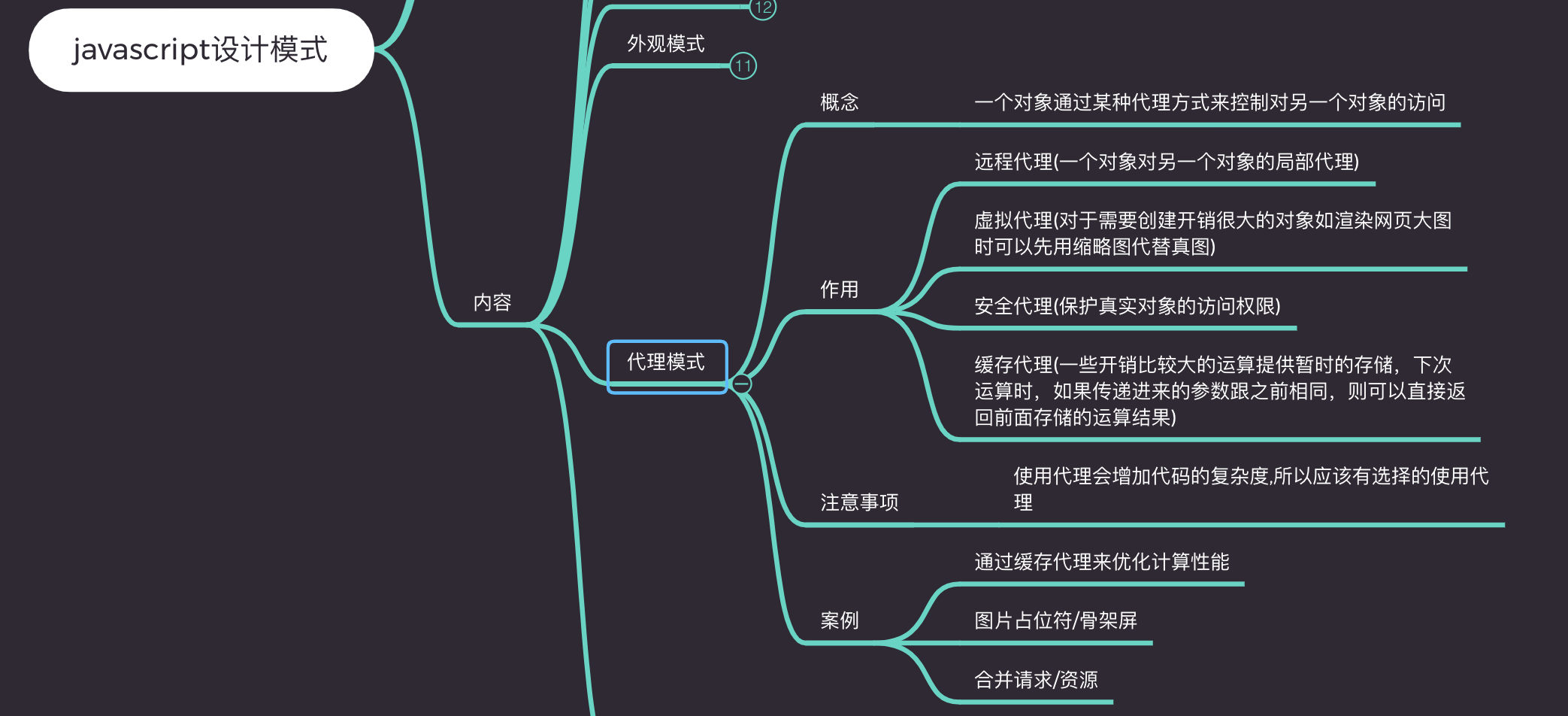#### 作用

• 远程代理(一个对象对另一个对象的局部代理)
• 虚拟代理(对于需要创建开销很大的对象如渲染网页大图时可以先用缩略图代替真图)
• 安全代理(保护真实对象的访问权限)
• 缓存代理(一些开销比较大的运算提供暂时的存储，下次运算时，如果传递进来的参数跟之前相同，则可以直接返回前面存储的运算结果)

#### 实际案例

• 通过缓存代理来优化计算性能
• 图片占位符/骨架屏/预加载等
• 合并请求/资源

#### 代码展示

``````// 缓存代理
function sum(a, b){
return a + b
}
let proxySum = (function(){
let cache = {}
return function(){
let args = Array.prototype.join.call(arguments, ',');
if(args in cache){
return cache[args];
}

cache[args] = sum.apply(this, arguments)
return cache[args]
}
})()``````

### 5. 外观模式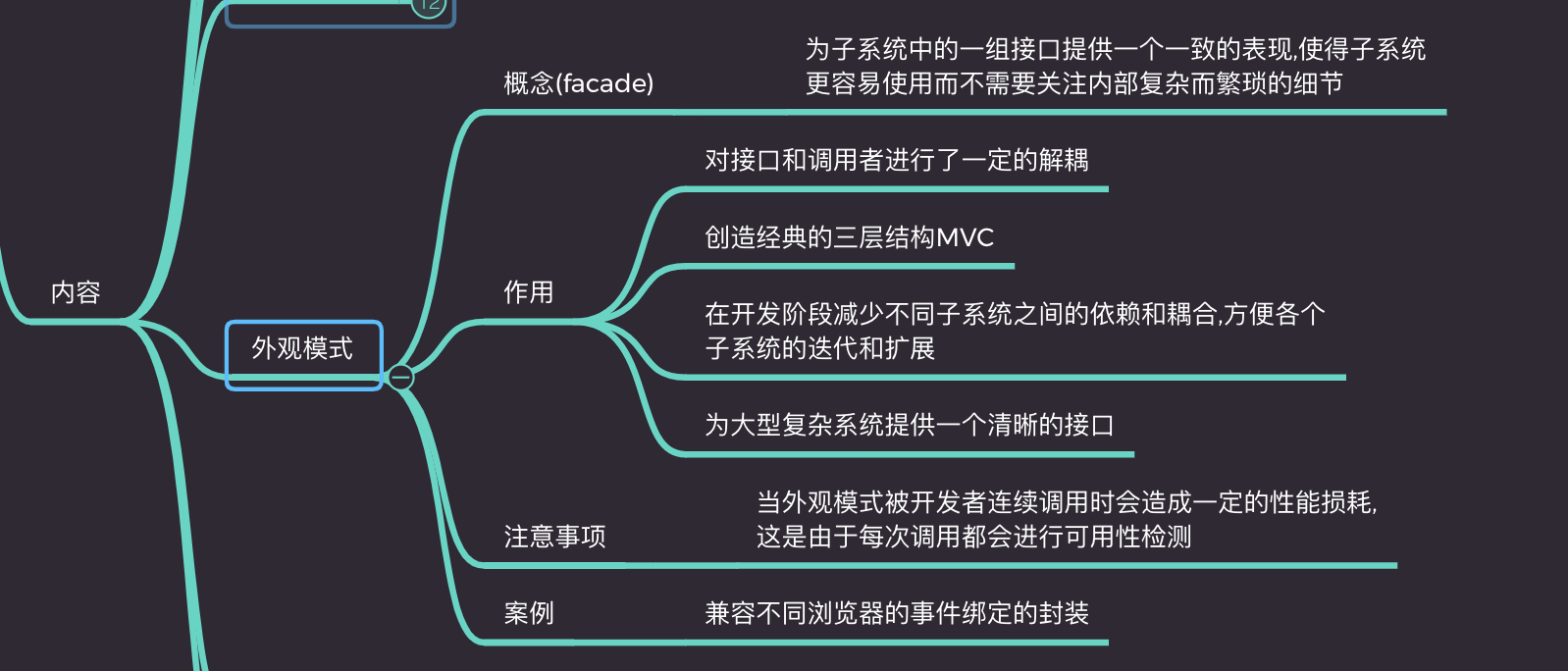#### 概念解读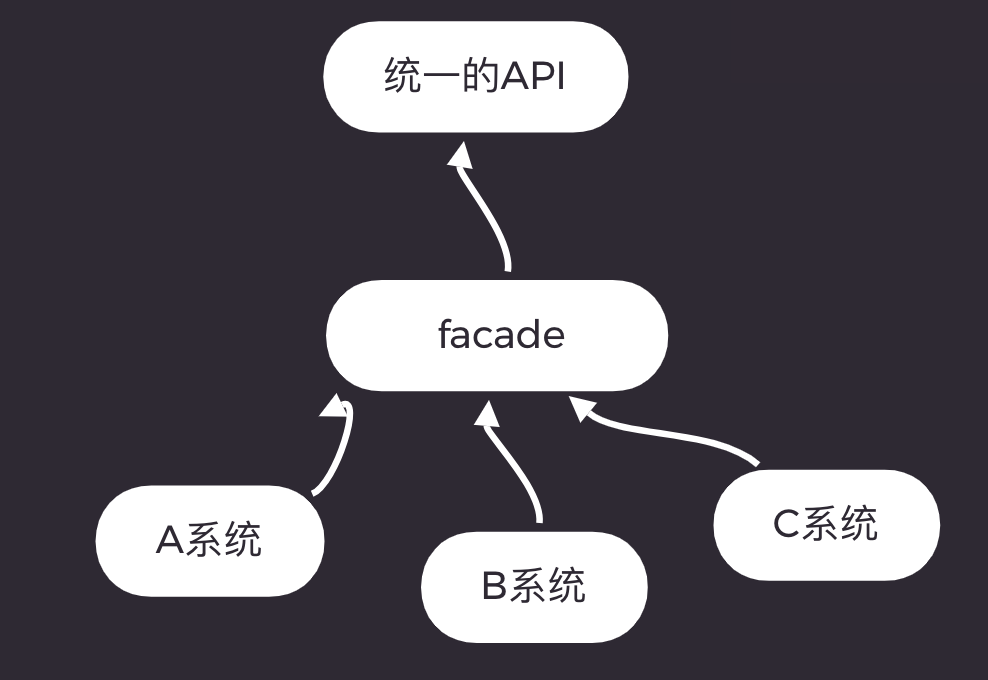#### 作用

• 对接口和调用者进行了一定的解耦
• 创造经典的三层结构MVC
• 在开发阶段减少不同子系统之间的依赖和耦合,方便各个子系统的迭代和扩展
• 为大型复杂系统提供一个清晰的接口

#### 代码展示

``````function on(type, fn){
// 对于支持dom2级事件处理程序
}else if(dom.attachEvent){
// 对于IE9一下的ie浏览器
dom.attachEvent('on'+type,fn);
}else {
dom['on'+ type] = fn;
}
}``````

### 6. 观察者模式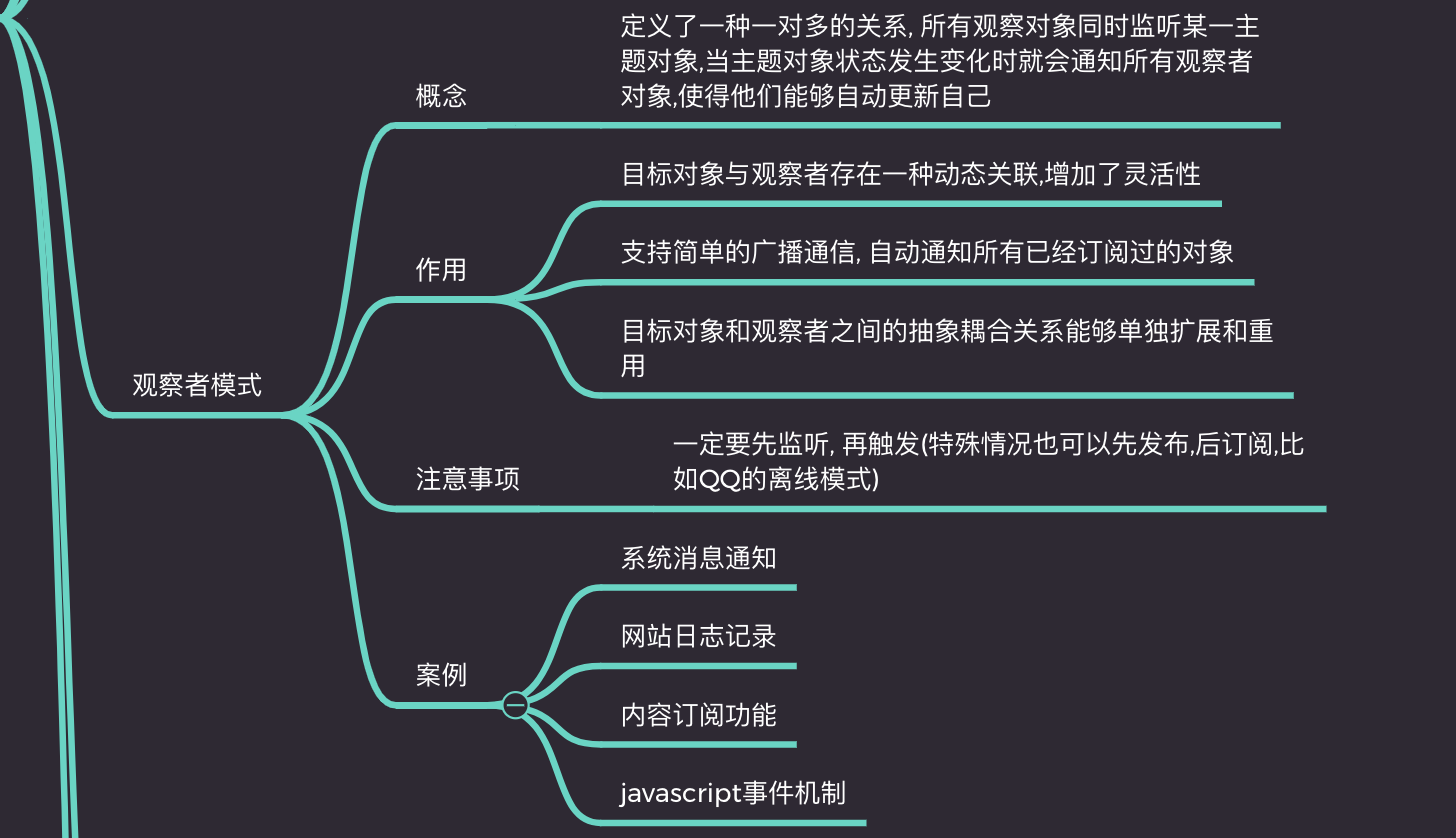#### 作用

• 目标对象与观察者存在一种动态关联,增加了灵活性
• 支持简单的广播通信, 自动通知所有已经订阅过的对象
• 目标对象和观察者之间的抽象耦合关系能够单独扩展和重用

#### 实际案例

• 系统消息通知
• 网站日志记录
• 内容订阅功能
• javascript事件机制
• react/vue等的观察者

#### 代码展示

``````class Subject {
constructor() {
this.subs = {}
}

const subArr = this.subs[key]
if (!subArr) {
this.subs[key] = []
}
this.subs[key].push(fn)
}

trigger(key, message) {
const subArr = this.subs[key]
if (!subArr || subArr.length === 0) {
return false
}
for(let i = 0, len = subArr.length; i < len; i++) {
const fn = subArr[i]
fn(message)
}
}

unSub(key, fn) {
const subArr = this.subs[key]
if (!subArr) {
return false
}
if (!fn) {
this.subs[key] = []
} else {
for (let i = 0, len = subArr.length; i < len; i++) {
const _fn = subArr[i]
if (_fn === fn) {
subArr.splice(i, 1)
}
}
}
}
}

// 测试
// 订阅
let subA = new Subject()
let A = (message) => {
console.log('订阅者收到信息: ' + message)
}

// 发布
subA.trigger('A', '我是徐小夕')   // A收到信息: --> 我是徐小夕``````

### 7. 策略模式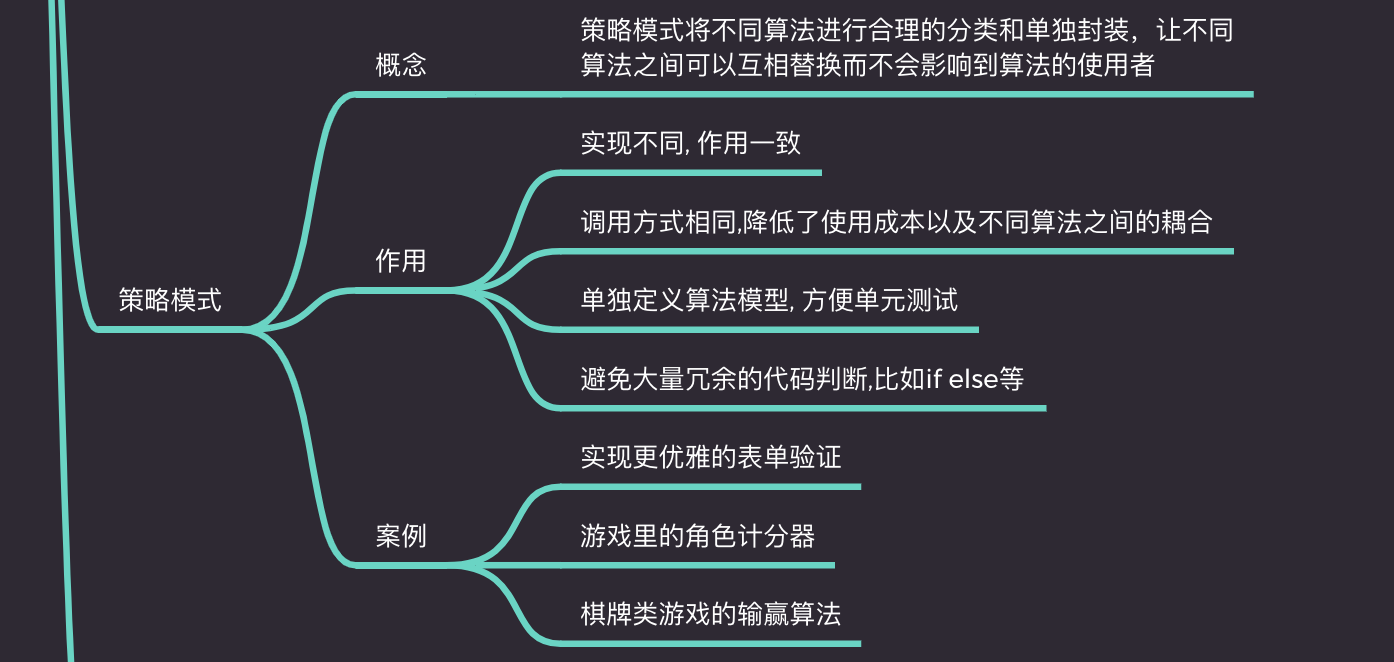#### 作用

• 实现不同, 作用一致
• 调用方式相同,降低了使用成本以及不同算法之间的耦合
• 单独定义算法模型, 方便单元测试
• 避免大量冗余的代码判断,比如if else等

• 实现更优雅的表单验证
• 游戏里的角色计分器
• 棋牌类游戏的输赢算法

#### 代码展示

``````const obj = {
A: (num) => num * 4,
B: (num) => num * 6,
C: (num) => num * 8
}

const getSum =function(type, num) {
return obj[type](num)
}``````

### 8. 迭代器模式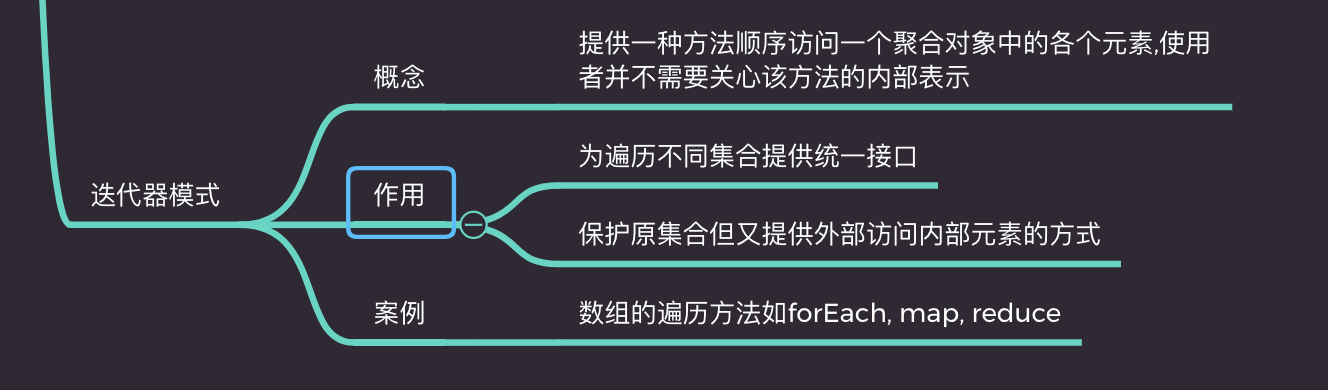#### 作用

• 为遍历不同集合提供统一接口
• 保护原集合但又提供外部访问内部元素的方式

#### 代码展示

``````function _each(el, fn = (v, k, el) => {}) {
// 判断数据类型
function checkType(target){
return Object.prototype.toString.call(target).slice(8,-1)
}

// 数组或者字符串
if(['Array', 'String'].indexOf(checkType(el)) > -1) {
for(let i=0, len = el.length; i< len; i++) {
fn(el[i], i, el)
}
}else if(checkType(el) === 'Object') {
for(let key in el) {
fn(el[key], key, el)
}
}
}``````

## 最后## 更多推荐

### 相关推荐

《css大法》之使用伪元素实现超实用的图标库（附源码

《前端实战总结》之使用解释器模式实现获取元素Xpath路径的算法

15分钟带你了解前端工程师必知的javascript设计模式(附详细思维导图和源码)

GoJS如何制作影像
JS 面相对象编程

AnyChart使用JavaScript构建箱形图
Canvas + JavaScript 制作图片粒子效果

GoJS用于HTML图表的JavaScript库